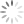Loading, please wait...# Integer and Float number representation

### 1) Integer Representation

Recall that an integer with the sign is called a signed integer. The signed integer has signs positive or negative. The signs are represented in the computer in the binary format as 1 for – (minus) and 0 for (plus) or vice versa. The sign bit is always coded as a leftmost bit for the representation of the sign of the number.

For example, positive signed numbers are represented in the form called signed magnitude form. In this form, the sign is represented by a binary 0 and the remaining magnitude by equivalent binary form.

+7 is represented as 0 0000111

The signed negative integers can be represented in any one of the following forms:

a) signed- magnitude form

b) signed- 1’s compliment form

c) signed- 2’s compliment form

In the signed magnitude form, the sign of the number is represented as I and the magnitude by equivalent binary form.

Example:

-7 is represented as 1 0000111

In the signed 1’s complement form, the sign of the integer is indicated by 1 and the magnitude is shown in 1’s complement form as follows,

-7 is represented as 1 1 1 1 1000

In the above signed -2’s complement form sign is indicated by 1 and magnitude by 2’s complement form as follows,

-7 is represented by 1 1111001

### 2) Floating Point Representation

In most of the application, a fraction is often used. The system of the number representation that keeps track of the position of the binary and decimal point is better than the fixed-point representation this system is called floating-point representation.

The real number is the integer part as well as the fractional part. The real number is also called the floating-point number. These numbers are either positive or negative. The real number 454.45 can be written 4.5445*102 or 0.45445*103. This type of representation of number is called the scientific representation. Using this scientific form, any number can be expressed as combination of mantissa and an exponent or we can say the number ‘n’ can be expressed as n=mre where m is the mantissa and r is the radix of the number system and e is the exponent. The mantissa is the fixed-point number. The exponent indicates the position of the binary or decimal point. For example the number 454.45

0.45445 3

mantissa Exponent

The zero in the leftmost position of the mantissa and exponent indicates the plus sign. The mantissa can be a fraction or integer depending upon make a computers use mantissa for fractional system representation.

Example in C:

1.2 E+ 12, 2.5E-2